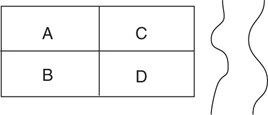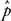# AP Statistics Practice Test 12

### Test Information10 questions23 minutes

1. A one-sided test of a hypothesis about a population mean, based on a sample of size 14, yields a P-value of 0.075. Which of the following best describes the range of tvalues that would have given this P-value?

2. Use the following excerpt from a random digits table for assigning six people to treatment and control groups:
98110 35679 14520 51198 12116 98181 99120 75540 03412 25631
The subjects are labeled: Arnold: 1; Betty: 2; Clive: 3; Doreen: 4; Ernie: 5; Florence: 6. The first three subjects randomly selected will be in the treatment group; the other three in the control group. Assuming you begin reading the table at the extreme left digit, which three subjects would be in the controlgroup?

3. A null hypothesis, H0: μ= μ0is to be tested against a two-sided hypothesis. A sample is taken; x- is determined and used as the basis for a C-level confidence interval (e.g., C= 0.95) for μ. The researcher notes that μ0is not in the interval. Another researcher chooses to do a significance test for μusing the same data. What significance level must the second researcher choose in order to guarantee getting the same conclusion about H0: μ= μ0(that is, reject or not reject) as the first researcher?

4. Which of the following is notrequired in a binomial setting?

5. Xand Yare independent random variables with μX= 3.5, μY= 2.7, σX= 0.8, and σY= 0.65. What are μX+Yand σX+Y?

6. A researcher is hoping to find a predictive linear relationship between the explanatory and response variables in her study. Accordingly, as part of her analysis she plans to generate a 95% confidence interval for the slope of the regression line for the two variables. The interval is determined to be (0.45, 0.80). Which of the following is (are) true? (Assume conditions for inference are met.)

I. She has good evidence of a linear relationship between the variables.

II. It is likely that there is a non-zero correlation (r) between the two variables.

III. It is likely that the true slope of the regression line is 0.

7. In the casino game of roulette, there are 38 slots for a ball to drop into when it is rolled around the rim of a revolving wheel: 18 red, 18 black, and 2 green. What is the probability that the first time a ball drops into the red slot is on the 8th trial (in other words, suppose you are betting on red every time-what is the probability of losing 7 straight times before you win the first time)?

8. You are developing a new strain of strawberries (say, Type X) and are interested in its sweetness as compared to another strain (say, Type Y). You have four plots of land, call them A, B, C, and D, which are roughly four squares in one large plot for your study (see the figure below). A river runs alongside of plots C and D. Because you are worried that the river might influence the sweetness of the berries, you randomly plant type Xin either A or B (and Yin the other) and randomly plant type Xin either C or D (and Yin the other). Which of the following terms best describes this design?9. Grumpy got 38 on the first quiz of the quarter. The class average on the first quiz was 42 with a standard deviation of 5. Dopey, who was absent when the first quiz was given, got 40 on the second quiz. The class average on the second quiz was 45 with a standard deviation of 6.1. Grumpy was absent for the second quiz. After the second quiz, Dopey told Grumpy that he was doing better in the class because they had each taken one quiz, and he had gotten the higher score. Did he really do better? Explain.

10. A random sample size of 45 is obtained for the purpose of testing the hypothesis H0: p= 0.80. The sample proportion is determined to be= 0.75. What is the value of the standard error offor this test?